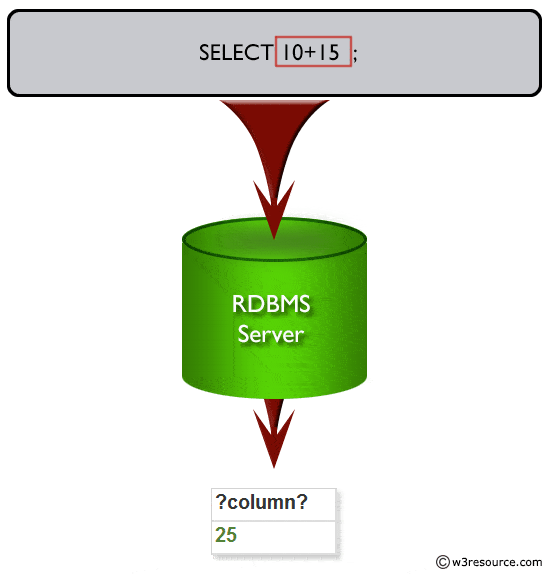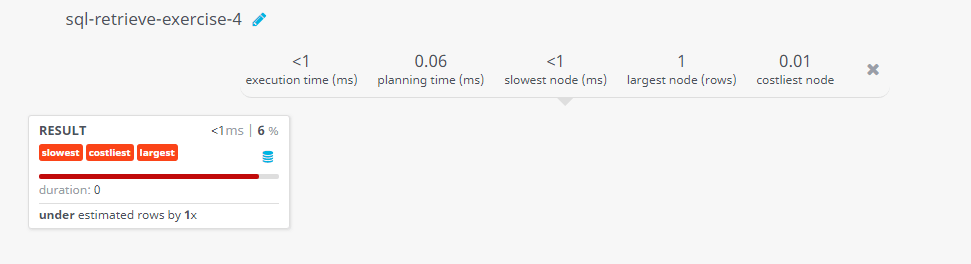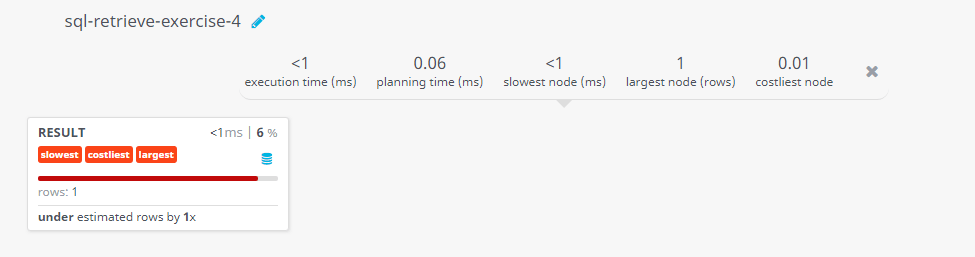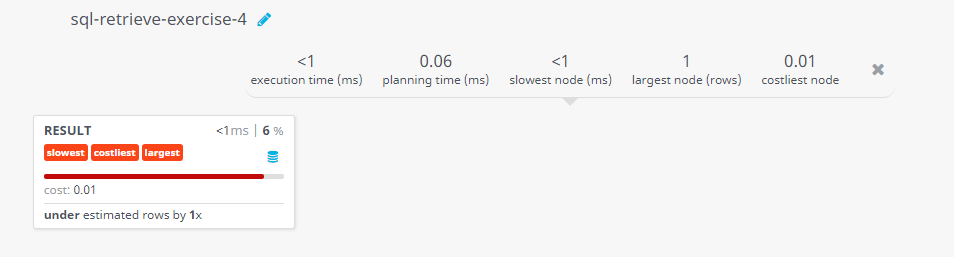﻿ SQL - Display the sum of two numbers

# SQL Exercises: Display the sum of two numbers

## SQL Basic Select Statement: Exercise-4 with Solution.

Write a SQL query to display the sum of two numbers 10 and 15 from the RDBMS server.

Sample Solution:

``````SELECT 10 + 15;
``````

Output of the Query:

```?column?
25```

Code Explanation:

The above SQL query that selects a column with a single row, which contains the result of the mathematical expression 10 + 15 = 25.Visual presentation:## Query Visualization:

Duration:Rows:Cost:Have another way to solve this solution? Contribute your code (and comments) through Disqus.

Previous SQL Exercise: Display the numbers in columns.
Next SQL Exercise: Display the result of an arithmetic expression.

What is the difficulty level of this exercise?

Test your Programming skills with w3resource's quiz.

﻿

## SQL: Tips of the Day

NOT IN vs NOT EXISTS

```SELECT ProductID,
ProductName
FROM   Products p
WHERE  NOT EXISTS (SELECT *
FROM   [Order Details] od
WHERE  p.ProductId = od.ProductId)
```

Ref: https://bit.ly/3Ez7mDx

We are closing our Disqus commenting system for some maintenanace issues. You may write to us at reach[at]yahoo[dot]com or visit us at Facebook